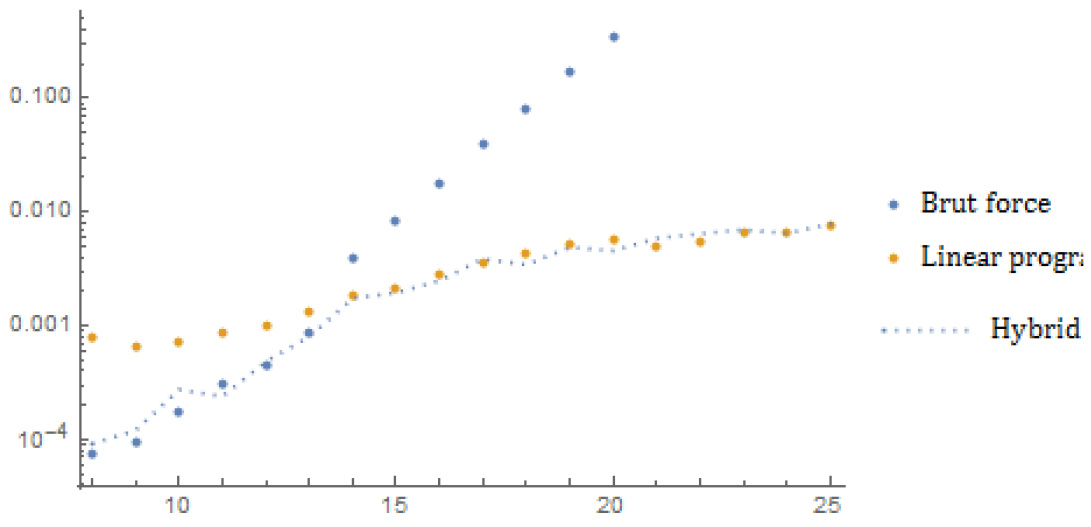#Wolfram Summer School

# Alumni# Xavier Roy

Summer School

Class of 2015

## Bio

Xavier Roy is a theoretical physicist. During his PhD and postdoc, he developed several mathematical models, algorithms and numerical simulations to describe and study perturbations in cosmology and the evolution of the universe.

## Project: The knapsack problem

The knapsack problem is a linear problem of combinatory optimization with constraints.

Given a set of n elements i of profits and costs ci, it consists in finding the solutions xi (the number of copies) that maximize the objective function ∑ pi xi while satisfying the constraint ∑ ci xi ⩽ C, with C a given parameter.

The 0-1 knapsack problem restricts the number of copies to 1 (an item i can be either picked (xi = 1) or not (xi = 0)), the bounded knapsack allows for a number of nc copies and the unbounded version for an unlimited number of copies.

Other possible extensions consider multiple objective functions ∑ pi xi → ∑ p ij xi), multiple constraints ( ∑ ci xi ⩽ C → ∑ cij xi ⩽ Cj) or combination of them.

The aim of the project is to explore the knapsack problem, implement in Mathematica an algorithm to solve it efficiently and define a user-friendly function from it.## Wolfram Summer School | Champaign, IL, USA | July 3 29–July 22, 2022

APPLY NOW
• 21st Annual Wolfram Summer School
• Bentley University, Waltham, MA, USA
• June 25–July 15, 2023
• Apply now